# FEBRUARY 2019

## Join me in February - in person or online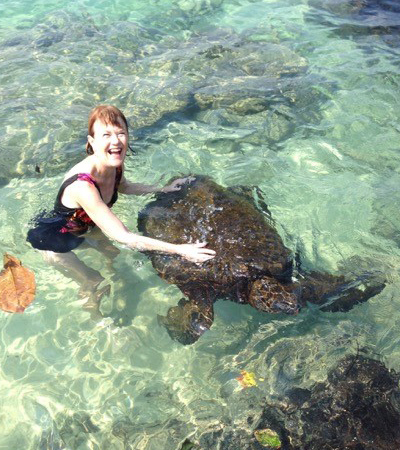Tanis in Hawaii.

Dear Friend,

Well I must say that the first few weeks of 2019 have been so exciting and wonderful - I do hope that you can say the same!

I launched my new book on the importance of ancestors, The High Beings of Hawaii: Encounters with mystical ancestors on January 15th. In a new video, I tell the surprising story of how this book came about.

Next week, I'll be taking part in the FREE Sacred Earth Telesummit, where I'll be speaking on celebration and co-creation with nature beings.

My workshop schedules for this year in both North America and Europe are now out and (mostly!) finalized.

And, finally, some photo highlights from my Hawaii workshops and book launch. Enjoy!

 table div table+table+table+table+table div table{width:100%;padding:0}table div table+table+table+table+table div table img{width:96.23%;padding:0;float:none}table div table+table+table+table+table div table td{width:100%;padding:0 1.88% 18px}/* styles *//* styles */
 table div table+table+table+table+table+table+table div table{width:100%;padding:0}table div table+table+table+table+table+table+table div table img{width:96.23%;padding:0;float:none}table div table+table+table+table+table+table+table div table td{width:100%;padding:0 1.88% 18px}/* styles */## NEW VIDEO: The High Beings of Hawaii

 /* styles */ I am so pleased to be able to tell you about my new book, The High Beings of Hawaii: Encounters with mystical ancestors! In this video, I tell the story of how the book came to be and my unexpected visit from my friend Lloyd the leprechaun while on vacation in Hawaii. Thank you so much to IIT member Verena Mezger, who filmed this video! Click on the image below to view on YouTube or try the direct link here: https://youtu.be/m2uL-msaOgo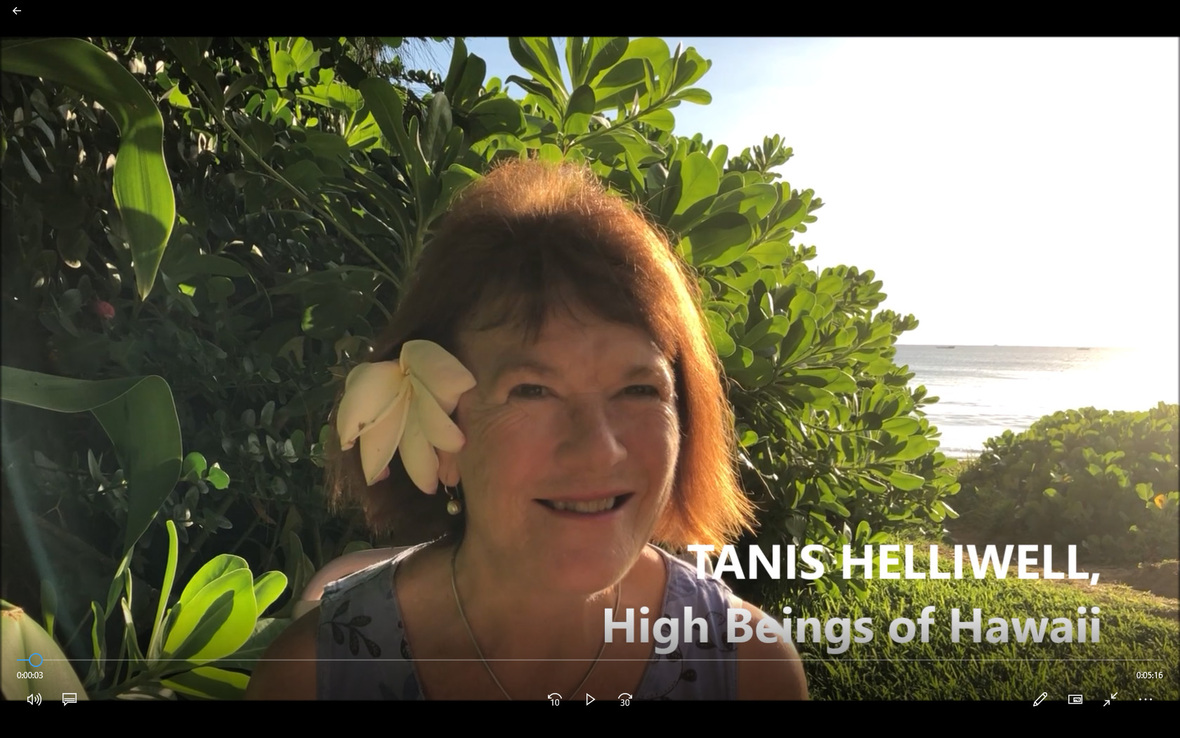Click on the image above to view the video on YouTube.
 table div table+table+table+table+table+table+table+table+table+table+table div table{width:100%;padding:0}table div table+table+table+table+table+table+table+table+table+table+table div table img{width:96.23%;padding:0;float:none}table div table+table+table+table+table+table+table+table+table+table+table div table td{width:100%;padding:0 1.88% 18px}/* styles */# Paperback & Kindle available NOW

 table div table+table+table+table+table+table+table+table+table+table+table+table+table+table div table,table.module-13{width:42.26%;float:left;padding:0}table div table+table+table+table+table+table+table+table+table+table+table+table+table+table div table a{border:0 none;text-decoration:none}table div table+table+table+table+table+table+table+table+table+table+table+table+table+table div table img{width:100%!important;border:0 none;text-decoration:none}table div table+table+table+table+table+table+table+table+table+table+table+table+table+table div table td{width:100%;padding:0 20px 20px 0}/* styles */ This Hawaiian vacation turns into a humorous, heart-opening odyssey that is alive with beings from other dimensions. Join Tanis’ adventure to meet seldom-seen beings of various races that dwell in volcanoes, misty forests and roaring seas. Discover the meaning behind our interconnected ancestral lineage. Receive practical tools from these physical and spiritual ancestors to heal the wounds in your life, in the lives of others and ultimately to help heal our wounded Earth. Follow the author along this meaningful path and you could develop a deep, intimate relationship with your own ancestors, elementals and mystical kin. This book is now available in English on Amazon.com (both paperback and Kindle), and will be available in March for the German edition.
 table div table+table+table+table+table+table+table+table+table+table+table+table+table+table+table+table+table div table{width:100%;padding:0}table div table+table+table+table+table+table+table+table+table+table+table+table+table+table+table+table+table div table img{width:96.23%;padding:0;float:none}table div table+table+table+table+table+table+table+table+table+table+table+table+table+table+table+table+table div table td{width:100%;padding:0 1.88% 18px}/* styles */# Praise for The High Beings of Hawaii:

“Tanis offers our Hawaiian ancestors the opportunity to speak to more people through her experiences with them and does this with humor and honor and captures their essence in her story.”

Kimokeo Kapahulehua, founder of Kimokeo Foundation preserving Native Hawaiian Culture

“A mystic and great storyteller, Tanis Helliwell … in describing her adventures on the Hawaiian Islands, captures the essence of what spiritual ancestors are all about. She weaves fascinating and enchanting tales of how she met the various old ones that reside there. You’ll be charmed and entertained as you read this book.”

Dr. Steven Farmer, author of Healing Ancestral Karma and Earth Magic

 table div table+table+table+table+table+table+table+table+table+table+table+table+table+table+table+table+table+table+table div table{width:100%;padding:0}table div table+table+table+table+table+table+table+table+table+table+table+table+table+table+table+table+table+table+table div table img{width:96.23%;padding:0;float:none}table div table+table+table+table+table+table+table+table+table+table+table+table+table+table+table+table+table+table+table div table td{width:100%;padding:0 1.88% 18px}/* styles */## FREE teleconference: Feb. 4-15

 table div table+table+table+table+table+table+table+table+table+table+table+table+table+table+table+table+table+table+table+table+table div table td,table.module-20{width:100%;padding:0}table div table+table+table+table+table+table+table+table+table+table+table+table+table+table+table+table+table+table+table+table+table div table{width:100%;float:none;margin-left:auto;margin-right:auto;padding:0}table div table+table+table+table+table+table+table+table+table+table+table+table+table+table+table+table+table+table+table+table+table div table a{border:0 none;text-decoration:none}table div table+table+table+table+table+table+table+table+table+table+table+table+table+table+table+table+table+table+table+table+table div table img{width:100%!important;border:0 none;text-decoration:none}/* styles */

I am excited to share with you a FREE event I'm going to be a part of: The Sacred Earth Telesummit next week. The purpose of this new multi-speaker event will show you how to:

▪ Create a nourishing connection to the Spirit of the Earth
▪ Take your place among the Beings of Nature
▪ Express the beauty of your own gifts
 ▪ Create a nourishing connection to the Spirit of the Earth
 ▪ Take your place among the Beings of Nature
 ▪ Express the beauty of your own gifts

There's no cost to attend, and even if you can't attend the talks live, you'll get access to each replay for 48 hours.

My talk will be on Monday. February 11 at 1:00 pm Pacific time. I will be speaking about "The Sacred Earth and You, a celebration and co-creation with nature beings". Join me for this talk and you will:

▪ Meet seldom-seen beings of various elemental and ancestral races that dwell in volcanoes, misty forests and roaring seas.
▪ Discover the meaning behind our interconnected ancestral lineage.
▪ Receive practical tools from these physical and spiritual ancestors to heal the wounds in your life, in the lives of others and ultimately to help heal our wounded Earth.
▪ Walk this meaningful path to develop a deep, intimate relationship with your own ancestors, elementals and mystical kin.
 ▪ Meet seldom-seen beings of various elemental and ancestral races that dwell in volcanoes, misty forests and roaring seas.
 ▪ Discover the meaning behind our interconnected ancestral lineage.
 ▪ Receive practical tools from these physical and spiritual ancestors to heal the wounds in your life, in the lives of others and ultimately to help heal our wounded Earth.
 ▪ Walk this meaningful path to develop a deep, intimate relationship with your own ancestors, elementals and mystical kin.

If you would like to learn how to create a deeper relationship with the Spirit of the Earth and the Beings of Nature, register today for the FREE Sacred Earth Telesummit.

 table div table+table+table+table+table+table+table+table+table+table+table+table+table+table+table+table+table+table+table+table+table+table+table+table div table{width:100%;padding:0}table div table+table+table+table+table+table+table+table+table+table+table+table+table+table+table+table+table+table+table+table+table+table+table+table div table img{width:96.23%;padding:0;float:none}table div table+table+table+table+table+table+table+table+table+table+table+table+table+table+table+table+table+table+table+table+table+table+table+table div table td{width:100%;padding:0 1.88% 18px}/* styles */## North America workshop schedule 2019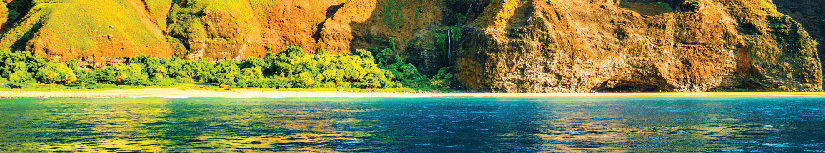/* styles */ I will be offering a Nature Spirits and Elementals workshop in Vancouver, Canada, in March. Check the listings and links below for up-to-the-minute updates.

# Events in North Vancouver, Canada in March:

## March 29: TALK: Elementals and Ancestors of Hawaii

Fee: \$20. To register or for information: Patricia pasmith321(at)gmail.com

## March 30-31: Co-creating with Nature Spirits to Heal the Earth

Early bird registration of \$150 and \$175 after Mar 1 with free attendance to Friday eve. Contact Patricia pasmith321(at)gmail.com

 table div table+table+table+table+table+table+table+table+table+table+table+table+table+table+table+table+table+table+table+table+table+table+table+table+table+table+table+table+table+table div table{width:100%;padding:0}table div table+table+table+table+table+table+table+table+table+table+table+table+table+table+table+table+table+table+table+table+table+table+table+table+table+table+table+table+table+table div table img{width:96.23%;padding:0;float:none}table div table+table+table+table+table+table+table+table+table+table+table+table+table+table+table+table+table+table+table+table+table+table+table+table+table+table+table+table+table+table div table td{width:100%;padding:0 1.88% 18px}/* styles */## Europe Workshop schedule 2019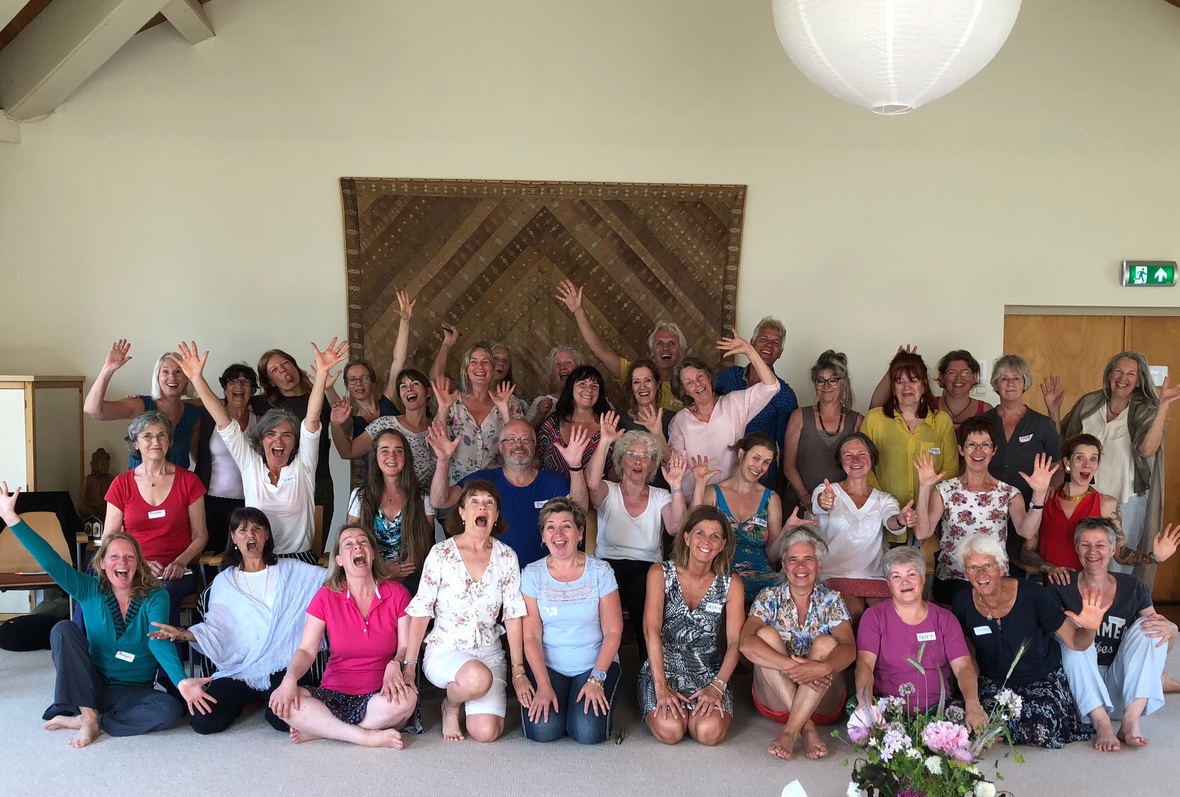/* styles */ My European workshop tour schedule for this spring/summer is now out! The focus this year is on: (1) Ancestors in Our Lives Today, and (2) Working with nature spirits and elementals. In addition, the European meditation retreat this June will assist you in self-healing with your body elemental. I'm so looking forward to these workshops, especially since I have just launched my new book, The High Beings of Hawaii: Encounters with mystical ancestors! In my talks on ancestors, I will discuss practical tools from these physical and spiritual ancestors to heal the wounds in your life, and in the lives of others. I do hope to see you soon!
 table div table+table+table+table+table+table+table+table+table+table+table+table+table+table+table+table+table+table+table+table+table+table+table+table+table+table+table+table+table+table+table+table+table+table+table div table{width:100%;padding:0}table div table+table+table+table+table+table+table+table+table+table+table+table+table+table+table+table+table+table+table+table+table+table+table+table+table+table+table+table+table+table+table+table+table+table+table div table img{width:96.23%;padding:0;float:none}table div table+table+table+table+table+table+table+table+table+table+table+table+table+table+table+table+table+table+table+table+table+table+table+table+table+table+table+table+table+table+table+table+table+table+table div table td{width:100%;padding:0 1.88% 18px}/* styles */## PHOTOS from Maui

 /* styles */ I've been having such a wonderful time meeting new people at my talks and workshops in Maui in January. I can't believe that my time here is almost up! Thank you so much to Theodor Carlat, who did an excellent job of organizing my talks and workshop. Here are some memorable moments that I'd like to share with you.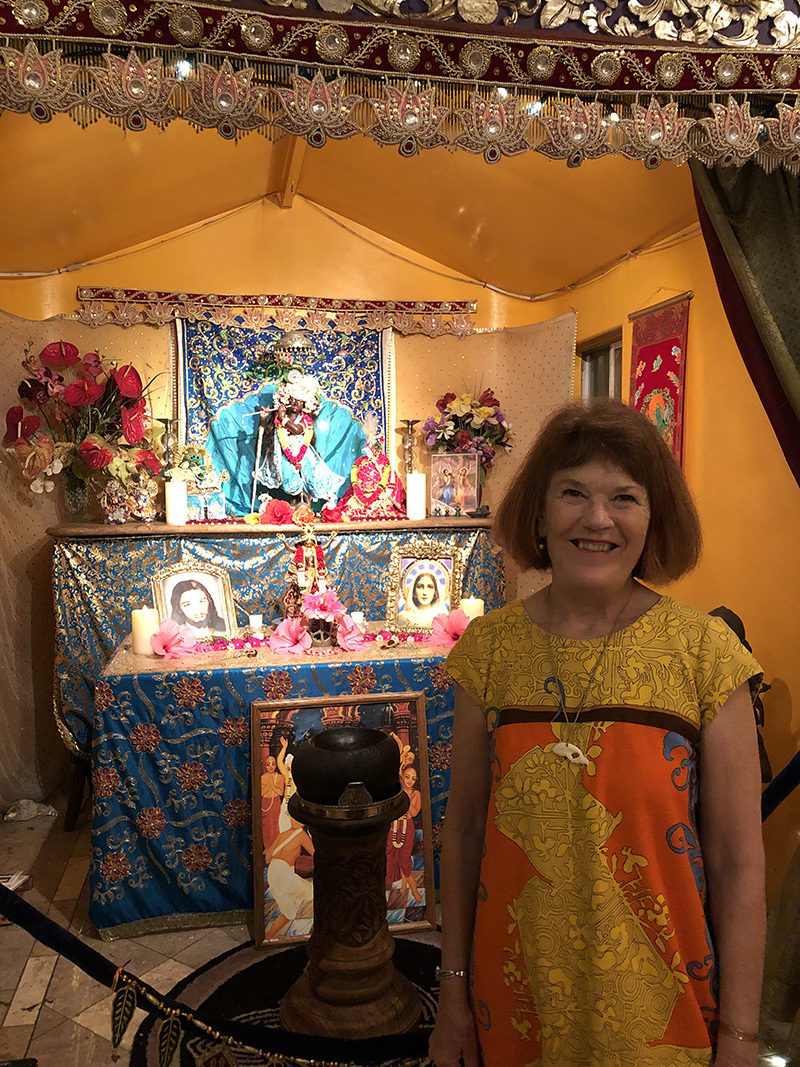Just returned from launching my new book, "The High Beings of Hawaii" at the Temple of Peace in Maui during a lunar eclipse. Absolutely magic.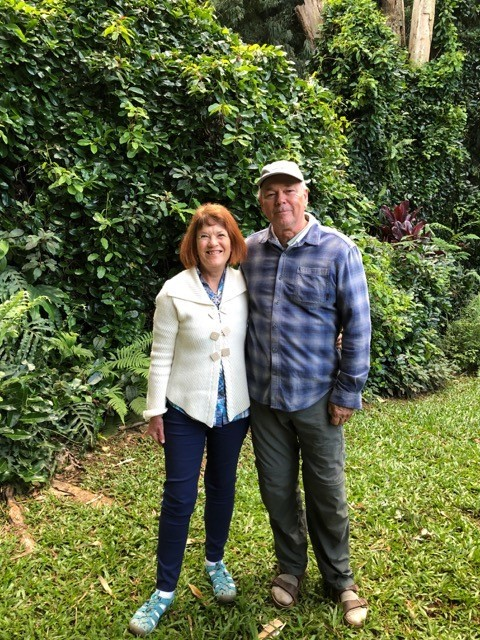Me with Maui workshop and talk organizer, Theodor Carlat.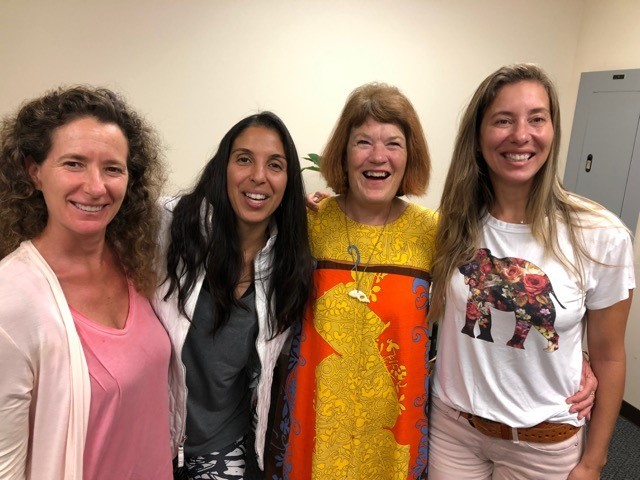Happy elemental lovers.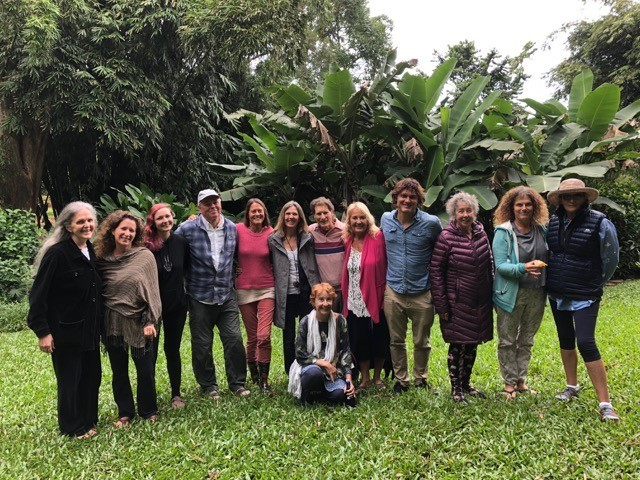Creating sanctuaries for nature spirits in Maui gardens.
 table div table+table+table+table+table+table+table+table+table+table+table+table+table+table+table+table+table+table+table+table+table+table+table+table+table+table+table+table+table+table+table+table+table+table+table+table+table+table+table+table+table div table{width:100%;padding:0}table div table+table+table+table+table+table+table+table+table+table+table+table+table+table+table+table+table+table+table+table+table+table+table+table+table+table+table+table+table+table+table+table+table+table+table+table+table+table+table+table+table div table img{width:96.23%;padding:0;float:none}table div table+table+table+table+table+table+table+table+table+table+table+table+table+table+table+table+table+table+table+table+table+table+table+table+table+table+table+table+table+table+table+table+table+table+table+table+table+table+table+table+table div table td{width:100%;padding:0 1.88% 18px}/* styles */## Send in your photos

 /* styles */ How sweet is this elaborate little fairy house? Found on Instagram at #fairygarden. Do you have an image of a leprechaun, gnome or another elemental? We would love to add it to the Leprechaun Website. Send it in to info(at)iitransform.com, or post it on Instagram using the hashtag #tanisleprechaun (remember to make it public) and we'll share it in our next IIT newsletter, too. I'm looking forward to seeing your photos.
 table div table+table+table+table+table+table+table+table+table+table+table+table+table+table+table+table+table+table+table+table+table+table+table+table+table+table+table+table+table+table+table+table+table+table+table+table+table+table+table+table+table+table+table+table div table td,table.module-43{width:100%;padding:0}table div table+table+table+table+table+table+table+table+table+table+table+table+table+table+table+table+table+table+table+table+table+table+table+table+table+table+table+table+table+table+table+table+table+table+table+table+table+table+table+table+table+table+table+table div table{width:100%;float:none;margin-left:auto;margin-right:auto;padding:0}table div table+table+table+table+table+table+table+table+table+table+table+table+table+table+table+table+table+table+table+table+table+table+table+table+table+table+table+table+table+table+table+table+table+table+table+table+table+table+table+table+table+table+table+table div table a{border:0 none;text-decoration:none}table div table+table+table+table+table+table+table+table+table+table+table+table+table+table+table+table+table+table+table+table+table+table+table+table+table+table+table+table+table+table+table+table+table+table+table+table+table+table+table+table+table+table+table+table div table img{width:100%!important;border:0 none;text-decoration:none}/* styles */
 table div table+table+table+table+table+table+table+table+table+table+table+table+table+table+table+table+table+table+table+table+table+table+table+table+table+table+table+table+table+table+table+table+table+table+table+table+table+table+table+table+table+table+table+table+table div table{width:100%;padding:0}table div table+table+table+table+table+table+table+table+table+table+table+table+table+table+table+table+table+table+table+table+table+table+table+table+table+table+table+table+table+table+table+table+table+table+table+table+table+table+table+table+table+table+table+table+table div table img{width:96.23%;padding:0;float:none}table div table+table+table+table+table+table+table+table+table+table+table+table+table+table+table+table+table+table+table+table+table+table+table+table+table+table+table+table+table+table+table+table+table+table+table+table+table+table+table+table+table+table+table+table+table div table td{width:100%;padding:0 1.88% 18px}/* styles *//* styles */ Love as always,/* styles */ Tanis Helliwell info@iitransform.com International Institute for Transformation
 Like   Tweet   Pin   +1